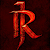# Beginning with Programming!

Programming is an art that cannot be learned in a year or so. You need to practice it continuously and regularly to be pro at it. But, before becoming a pro, some basic fundamentals and foundations are important. …

# Make Your Python Code Faster

I’m sure you heard a lot of people complaining that Python is so slow. I see people compare Python to C in the context of performance only, but they don’t compare in the context of fast development.

It is a dynamically-typed language meaning its variable types are not predefined, although…

# Naive Solution

The first approach for this problem that comes to mind is to sort the array. Thus approach will work in O(nlogn) if we sorting techniques like merge sort. So that’s the naive solution to this problem.

`class Solution:    def sortColors(self, nums: List[int]) -> None:        nums.sort()`

Time Complexity: O(nlogn)

Space Complexity…

# Day 9: Jump Game VI

Problem Statement:

You are given a 0-indexed integer array `nums` and an integer `k`.

You are initially standing at index `0`. In one move, you can jump at most `k` steps forward without going outside the boundaries of the array. …

# Day 8: Construct Binary Tree from Preorder and Inorder Traversal

Problem Statement:

Given two integer arrays `preorder` and `inorder` where `preorder` is the preorder traversal of a binary tree and `inorder` is the inorder traversal of the same tree, construct and return the binary tree.

Example 1:

`Input: preorder = [3,9,20,15,7], inorder = [9,3,15,20,7]Output: [3,9,20,null,null,15,7]`

Example 2:

`…`

# Day 7: Min Cost Climbing Stairs

Problem Statement:

You are given an integer array `cost` where `cost[i]` is the cost of `ith` step on a staircase. Once you pay the cost, you can either climb one or two steps.

You can either start from the step with index `0`, or the step with index…

# Day 6: Longest Consecutive Sequence

Problem Statement:

Given an unsorted array of integers `nums`, return the length of the longest consecutive elements sequence.

You must write an algorithm that runs in `O(n)` time.

Example 1:

`Input: nums = [100,4,200,1,3,2]Output: 4Explanation: The longest consecutive elements sequence is [1, 2, 3, 4]. …`

# Day 5: Maximum Performance of a Team

Problem Statement:

You are given two integers `n` and `k` and two integer arrays `speed` and `efficiency` both of length `n`. There are `n` engineers numbered from `1` to `n`. `speed[i]` and `efficiency[i]` represent the speed and efficiency of the `ith` engineer respectively.

Choose at most `k` different…

# Day 4: Open the Lock

Problem Statement:

You have a lock in front of you with 4 circular wheels. Each wheel has 10 slots: `'0', '1', '2', '3', '4', '5', '6', '7', '8', '9'`. The wheels can rotate freely and wrap around: for example, we can turn `'9'` to `'0'`, or `'0'` to…

# Day 3: Maximum Area of a Piece of Cake After Horizontal and Vertical Cuts

Given a rectangular cake with height `h` and width `w`, and two arrays of integers `horizontalCuts` and `verticalCuts` where `horizontalCuts[i]` is the distance from the top of the rectangular cake to the `ith` horizontal cut and similarly, `verticalCuts[j]` is the distance from the left of…